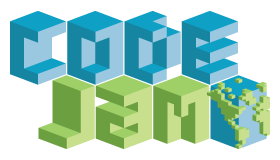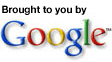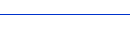Important Dates Registration Starts Monday, August 14th Qualification Round Tuesday, September 5th Championship Round Friday, October 27th\$165,000 inCash & Prizes! 1st Place - \$10,000 2nd - 10th Place - \$5,000 11th - 25th Place - \$2,500 26th - 50th Place - \$1,000 51st - 100th Place - \$750
TopCoder C++ for C Programmers

C++ is an object oriented extension to the C programming language. C++ provides many benefits over traditional C while still maintaining C compatibility, allowing people to use C and C++ program in the same program. Within a competition, you'll be required to use some basic C++ functionality to sovle problems. What follows is a basic explanation for programmers who are currently familiar with C.

Classes and Methods
The most important new feature in C++ is the addition of classes. A class is a structure that contains methods as well as variables. During a competition, you'll need to write one class containing (at least) one method. For example, take the following problem definition:

Class: CellTower
Method: best
Parameters: vector <string>, int, int
Returns: int
Method signature: int best(vector <string> towers, int x, int y)

To solve the problem you'll need to create a class named CellTower containing a method best that takes three arguements (vector<string>, int, and int), and returns an int.

Classes are defined using the class keyword. The definition follows a similar pattern as the C struct keyword. To define the class above we'd use the following code:
```             class CellTower {
public:
int best(vector<string> towers, int x, int y) {
}
};
```
Note how the method is defined like you would define a standard C function. The public keyword tells the compiler that the method we're defining is accessable to any object, allowing the testing process to properly execute your code.

The STL
Many of the classes and functions used in competition come from the Standard Type Library, also known as the STL. The STL provides a set of common libraries to perform everything from basic string work to complicated sorting algorithms.

To be able to compete, you'll need to be familiar with two classes: vector and string.

Includes
Before you can use any of the STL classes, you'll need to include the appropriate header files. The vector class comes from the header "vector" and the string class comes from the header "string". In addition, you'll need to add the line
`using namespace std;`
to your code to tell the compiler to look for objects in the std namespace.

Vector
A vector is the C++ replacement for arrays. Vectors solve many of the problems of tradidional C arrays by allowing dynamic resizing and providing methods to inspect the current size of the array. You declare a vector as vector<type> where type is the type of variable stored in the array. To create a vector of ints, you'd write
`vector<int> myVar;`
Newly created vectors are of size 0. To declare a vector with a specific size, you can use
```vector<int>
myVar(10);```
In this case the newly created vector has a size of 10.

To set / retrieve the elements in a vector, you can use the same syntax you'd use to work with a C array.
```             vector<int> myVar(10);

myVar = 1; //sets the first element to 1
printf("%i", myVar); //prints 1
```
One of the major problems with C arrays is that there is no way at runtime to know how large the array is, making looping over the contents of the array difficult. Using vectors, this task becomes simple. The size() method will return the current size of the vector.
```             for(int i = 0; i < myVar.size(); i++) {
printf("%i", myVar[i]); //prints element i
}
```
To resize a vector, use the resize method.
```             myVar.resize(15); //sets the size of myVar to 15
```

String
The string class is designed to replace using the char* type to represent text. Strings allow for basic manipulation, and provides the ability to use the string class in functions that require a char*. To assign values to a string, you can use the = and + operators.
```             string s;
s = "Hello";
s = s + ", world";
```
The string s is initially created as an empty string (a string of size zero), is assigned the value "Hello", then has ", world" appended to the end of the string. The string s now contains "Hello, world".

The method size() will return the length of the string. The code
```             string s = "Hello";
printf("%i", s.size());
```
will output 5.

You can access specific characters in the string by using the object as if it were a char array.
```             string s = "Hello";
printf("%c", s); //outputs "H"
s = 'h'; //string is now "hello"
```
To use the string in a function that expects a char*, use the c_str() method.
```             string s = "Hello";
printf("%s", s.c_str()); //outputs "Hello"
```
Example Submission
Consider the following sample problem:

Our input program has recorded keyboard input into an array of characters. We want to convert the input sequence to a single string for further processing. Make a method buildString that takes a vector<char> representing the keyboard input and returns a concatenated string.

Class: StringBuilder
Method: buildString
Parameters: vector <char>
Returns: string
Method signature: string buildString(vector <char> array)

The following submission would use the string and vector classes to solve the problem:
```             #include<vector>
#include<string>

using namespace std;

class StringBuilder {
public:
string buildString(vector<char> array) {
//create string for return value
string s;

//loop over every element in the array
for(int i = 0; i < array.size(); i++) {
s = s + array[i];
}

//return the created string
return s;
}
};
```
The C++ and STL libraries contains hundreds of functions and classes designed to increase coding speed and efficiency. The references below should provide a good overview of other capabilities of the objects discussed, and C++ in general.

C++:
C++ Tutorial
C++ Tutorial for C users

Namespaces:
Namespace Tutorial
C++ Namespaces

Vectors:
Vector Reference
The Vector Class

STL:
STL Programmer's Guide
A Crash Course in the C++ Standard Template Library